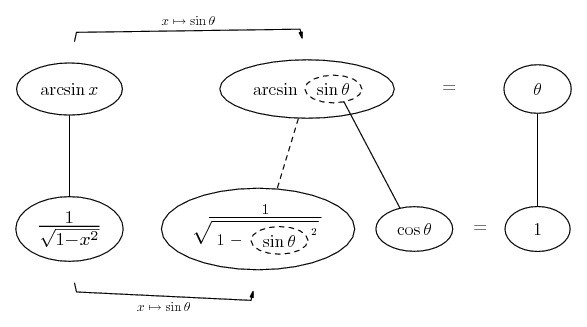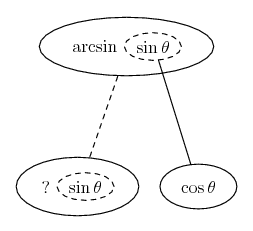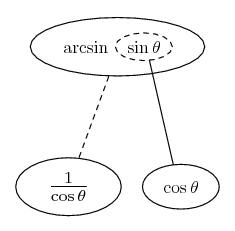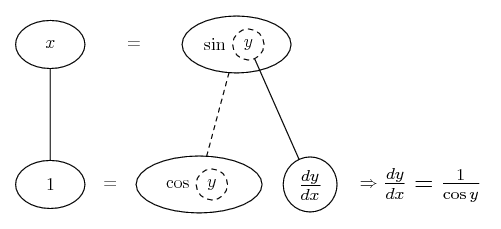# Thread: trigonometry

1. ## trigonometry

it is given that y=sin^-1,that is, y=arc sinx. by expressing x as a function of y, determine dx/dy and hence determine dy/dx as a function of x only2.Originally Posted by Rose Wanjohiit is given that y=sin^-1,that is, y=arc sinx. by expressing x as a function of y, determine dx/dy and hence determine dy/dx as a function of x onlyNote that $\displaystyle y=\arcsin x\implies\sin y =x$

Now, differentiate w.r.t. y to get $\displaystyle \cos y=\frac{\,dx}{\,dy}$.

From here, it's evident that $\displaystyle \frac{\,dy}{\,dx}=\frac{1}{\cos y}$

I leave it for you to show that if $\displaystyle \sin y=x$, then $\displaystyle \cos y = \sqrt{1-x^2}$.

Thus, it follows that if $\displaystyle y=\arcsin x$, then $\displaystyle \frac{\,dy}{\,dx}=\frac{1}{\cos y}=\frac{1}{\sqrt{1-x^2}}$

Does this make sense?

3.Originally Posted by Rose Wanjohiit is given that y=sin^-1,that is, y=arc sinx. by expressing x as a function of y, determine dx/dy and hence determine dy/dx as a function of x only$\displaystyle y=arcsin x$

$\displaystyle x=sin y$ -------- (1)

$\displaystyle \frac{dx}{dy}=cos y$

$\displaystyle \frac{dy}{dx}=\frac{1}{cos y}$

Using (1), $\displaystyle cos^2y=1-x^2$

$\displaystyle \frac{dy}{dx}=\frac{1}{\sqrt{1-x^2}}$

4. Just in case a picture helps...This isn't quite to express x as a function of y - I'll put a balloon version of that further down the post (later)... but this here is the picture I find best of all for this problem. .

As usual, straight continuous lines differentiate downwards (integrate up) with respect to the explicit variable (x or theta) and the straight dashed line similarly but with respect to the dashed balloon expression.

For enlightenment, work from the top left corner - the given expression arcsin x. Then consider arcsin as the outer function of a chain rule differentiation...If the inner function is sin, then the derivative with respect to sin will be multiplied by the derivative of sin...But then arcsin(sin(theta)) is of course theta, and the derivative of that is 1, so the whole of the bottom of the chain-rule shape wants to come to 1, so we know it must be...Which we just re-write using Pythag and then - swapping sin theta back for x - we finish at the bottom left corner...

_______________________________

And if you do need to express x as a function of y...... and proceed as per the other 2 posts.

Don't integrate - balloontegrate!

Balloon Calculus Forum

#### Search Tags

trigonometry